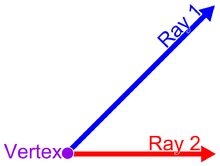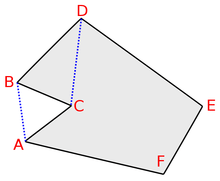# Vertex (geometry)

For vertices in the geometry of curves, see Vertex (curve). For other uses of the word, see Vertex (disambiguation).

In geometry, a vertex (plural: vertices or vertexes) is a point where two or more curves, lines, or edges meet. As a consequence of this definition, the point where two lines meet to form an angle and the corners of polygons and polyhedra are vertices.

## Definition

### Of an angleA vertex of an angle is the endpoint where two line segments or rays come together.

The vertex of an angle is the point where two rays begin or meet, where two line segments join or meet, where two lines intersect (cross), or any appropriate combination of rays, segments and lines that result in two straight "sides" meeting at one place.

### Of a polytope

A vertex is a corner point of a polygon, polyhedron, or other higher-dimensional polytope, formed by the intersection of edges, faces or facets of the object.

In a polygon, a vertex is called "convex" if the internal angle of the polygon, that is, the angle formed by the two edges at the vertex, with the polygon inside the angle, is less than π radians ( 180°, two right angles) ; otherwise, it is called "concave" or "reflex". More generally, a vertex of a polyhedron or polytope is convex if the intersection of the polyhedron or polytope with a sufficiently small sphere centered at the vertex is convex, and concave otherwise.

Polytope vertices are related to vertices of graphs, in that the 1-skeleton of a polytope is a graph, the vertices of which correspond to the vertices of the polytope, and in that a graph can be viewed as a 1-dimensional simplicial complex the vertices of which are the graph's vertices. However, in graph theory, vertices may have fewer than two incident edges, which is usually not allowed for geometric vertices. There is also a connection between geometric vertices and the vertices of a curve, its points of extreme curvature: in some sense the vertices of a polygon are points of infinite curvature, and if a polygon is approximated by a smooth curve there will be a point of extreme curvature near each polygon vertex. However, a smooth curve approximation to a polygon will also have additional vertices, at the points where its curvature is minimal.

### Of a plane tiling

A vertex of a plane tiling or tessellation is a point where three or more tiles meet; generally, but not always, the tiles of a tessellation are polygons and the vertices of the tessellation are also vertices of its tiles. More generally, a tessellation can be viewed as a kind of topological cell complex, as can the faces of a polyhedron or polytope; the vertices of other kinds of complexes such as simplicial complexes are its zero-dimensional faces.

## Principal vertexVertex B is an ear, because the open line segment between C and D is entirely inside the polygon. Vertex C is a mouth, because the open line segment between A and B is entirely outside the polygon.

A polygon vertex xi of a simple polygon P is a principal polygon vertex if the diagonal [x(i − 1), x(i + 1)] intersects the boundary of P only at x(i − 1) and x(i + 1). There are two types of principal vertices: ears and mouths.

### Ears

A principal vertex xi of a simple polygon P is called an ear if the diagonal [x(i − 1), x(i + 1)] that bridges xi lies entirely in P. (see also convex polygon) According to the two ears theorem, every simple polygon has at least two ears.

### Mouths

A principal vertex xi of a simple polygon P is called a mouth if the diagonal [x(i − 1), x(i + 1)] lies outside the boundary of P.

## Number of vertices of a polyhedron

Any convex polyhedron's surface has Euler characteristicwhere V is the number of vertices, E is the number of edges, and F is the number of faces. This equation is known as Euler's polyhedron formula. Thus the number of vertices is 2 more than the excess of the number of edges over the number of faces. For example, a cube has 12 edges and 6 faces, and hence 8 vertices.

## Vertices in computer graphics

In computer graphics, objects are often represented as triangulated polyhedra in which the object vertices are associated not only with three spatial coordinates but also with other graphical information necessary to render the object correctly, such as colors, reflectance properties, textures, and surface normals; these properties are used in rendering by a vertex shader, part of the vertex pipeline.

## References

1. Heath, Thomas L. (1956). The Thirteen Books of Euclid's Elements (2nd ed. [Facsimile. Original publication: Cambridge University Press, 1925] ed.). New York: Dover Publications.
(3 vols.): ISBN 0-486-60088-2 (vol. 1), ISBN 0-486-60089-0 (vol. 2), ISBN 0-486-60090-4 (vol. 3).
2. Jing, Lanru; Stephansson, Ove (2007). Fundamentals of Discrete Element Methods for Rock Engineering: Theory and Applications. Elsevier Science.
3. Peter McMullen, Egon Schulte, Abstract Regular Polytopes, Cambridge University Press, 2002. ISBN 0-521-81496-0 (Page 29)
4. Bobenko, Alexander I.; Schröder, Peter; Sullivan, John M.; Ziegler, Günter M. (2008). Discrete differential geometry. Birkhäuser Verlag AG. ISBN 978-3-7643-8620-7.
5. M.V. Jaric, ed, Introduction to the Mathematics of Quasicrystals (Aperiodicity and Order, Vol 2) ISBN 0-12-040602-0, Academic Press, 1989.
6. Devadoss, Satyan; O'Rourke, Joseph (2011). Discrete and Computational Geometry. Princeton University Press. ISBN 978-0-691-14553-2.
7. Meisters, G. H. (1975), "Polygons have ears", The American Mathematical Monthly, 82: 648–651, doi:10.2307/2319703, MR 0367792.
8. Christen, Martin. "Clockworkcoders Tutorials: Vertex Attributes". Khronos Group. Retrieved 26 January 2009.